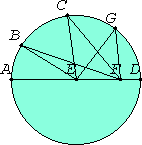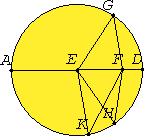# Proposition 7

If on the diameter of a circle a point is taken which is not the center of the circle, and from the point straight lines fall upon the circle, then that is greatest on which passes through the center, the remainder of the same diameter is least, and of the rest the nearer to the straight line through the center is always greater than the more remote; and only two equal straight lines fall from the point on the circle, one on each side of the least straight line.

Let ABCD be a circle, and let AD be a diameter of it. Let F be a point F on AD which is not the center of the circle. Let E be the center of the circle. Let straight lines FB, FC, and FG fall upon the circle ABCD from F.

I say that FA is greatest, FD is least, and of the rest FB is greater than FC, and FC greater than FG.Join BE, CE, and GE.

I.20

Then, since in any triangle the sum of any two sides is greater than the remaining one, the sum of EB and EF is greater than BF.

But AE equals BE, therefore AF is greater than BF.

I.24

Again, since BE equals CE, and FE is common, the two sides BE and EF equal the two sides CE and EF. But the angle BEF is also greater than the angle CEF, therefore the base BF is greater than the base CF.

For the same reason CF is also greater than GF.

I.20

Again, since the sum of GF and FE is greater than EG, and EG equals ED, the sum of GF and FE is greater than ED.

Subtract EF from each. Therefore the remainder GF is greater than the remainder FD.

Therefore FA is greatest, FD is least, FB is greater than FC, and FC greater than FG.

I say also that from the point F only two equal straight lines fall on the circle ABCD, one on each side of the least FD.

I.23

Construct the angle FEH equal to the angle GEF on the straight line EF and at the point E on it. Join FH.I.4

Then, since GE equals EH, and EF is common, the two sides GE and EF equal the two sides HE and EF, and the angle GEF equals the angle HEF, therefore the base FG equals the base FH.

I say again that another straight line equal to FG does not fall on the circle from the point F.

For, if possible, let FK so fall.

Above

Then, since FK equals FG, and FH equals FG, FK also equals FH, the nearer to the straight line through the center being thus equal to the more remote, which is impossible.

Therefore another straight line equal to GF does not fall from the point F upon the circle. Therefore only one straight line so falls.

Therefore if on the diameter of a circle a point is taken which is not the center of the circle, and from the point straight lines fall upon the circle, then that is greatest on which passes through the center, the remainder of the same diameter is least, and of the rest the nearer to the straight line through the center is always greater than the more remote; and only two equal straight lines fall from the point on the circle, one on each side of the least straight line.

Q.E.D.

## Guide

The statement of this proposition is daunting. It concerns the distances from a point F inside a circle to the points on the circumference. The point F is assumed not to be the center. If a diameter AD is passed through F, then one of the points A is the point on the circumference furthest from F and the other D is the closest. As a point travels the circumference from A to D it gets closer to F. The final part of the statement is that if G is one point on the circumference, then there is exactly one other point H on the circumference the same distance from F (assuming, of course, that G is neither A nor D).

#### Note

There is some confusion in the statement of this proposition. It is not clear what the phrase “the nearer to the straight line through the center” means. Perhaps it refers to the angle, so that FB is considered nearer to FA than FC since the angle BFA is less than the angle CFA. If so, there there is a missing detail in the proof, as DeMorgan pointed out. It is declared that the angle BEF is greater than the angle CEF, but that hasn’t been proved. DeMorgan and others have described various ways to fill this logical gap.

This proposition is not used in the rest of the Elements.